# To Be “Continued” — an Unusual Fraction

Here is an interesting fraction to ponder: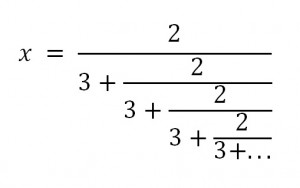Constructions such as these are called “continued fractions”. Though they go on forever, there is a way to evaluate them.  Before we do that, let’s get a feel for the answer we seek by figuring out an upper and lower limit on the value.

1.  x is less than 2/3.

We know this because the numerator is 2 and the denominator is 3 + something positive.  If the denominator is MORE than 3, then the fraction is LESS than 2/3.  But how much less?  Well, there is a limit on that as well…

2. x is more than 6/11.

As we just said, the denominator is more than 3, but how much more?  Look again at the “something positive” which is added to the denominator:  it is in fact the same expression x that we are hoping to find.  We don’t know its value, but we know it is less than 2/3.  So now we have: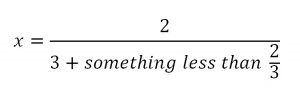The fraction will have its smallest value when the denominator is the biggest it can be.

That gives us a lower limit on the value of x: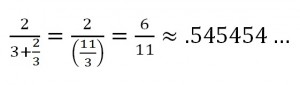But what if we want an exact value?  It turns out that we’ve already noticed the key to this puzzle.

Look again at the continued fraction.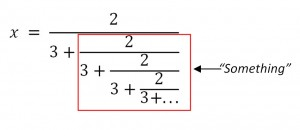The denominator is more than 3…but notice just how much more:  the expression added to 3 is the same as the original expression, x.

That means we can re-write the continued fraction this way:We multiply both sides by 3+x and distribute on the left hand side to get: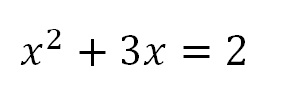Were you expecting that?  To evaluate the continued fraction, we have to solve a quadratic equation.  This means that we are going to have to choose a method.  I’m going to vote for completing the square (which I will return to in my next post).  But if you prefer, you can use the quadratic formula.  Either way, you’ll get: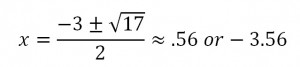It’s  the positive answer that we want here.  And its nice to see that it falls in the range we expected.

I still like completing the square better.  Before we get to that, here’s one more fun example that will end with a twist.

THE GOLDEN CONTINUED FRACTION: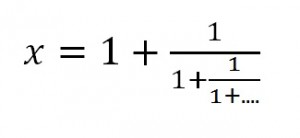Let’s call it an exercise (or two):

Show that x must be less than 2 and more than 1.5.

(You can post your reasoning in a comment if you like.)

But again, what if we want the exact value?  We have to notice that once again, the expression we seek is hidden inside the continued fraction. Here it is again: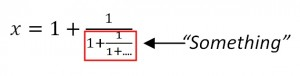Look closely – that “something” is the same expression we started with.  It’s x!

So we can re-write the original equation:And if we multiply both sides by x:Or: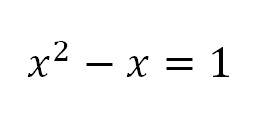We still have to solve this quadratic equation.  But those (both?) of you who have been following along with me might remember that we have seen this equation before.  This was exactly the quadratic equation we landed on when looking for the Golden Ratio.  The one equation answers two seemingly unrelated questions:

1. What is the value of this expression with a continued fraction?2. What is the aspect ratio of a rectangle from which you can remove a square, leaving behind a smaller rectangle with the same aspect ratio?

We’ll know both of these when we solve that quadratic equation.

(That was my idea of a cliff-hanger ending.)

“I was never any good in sports.  I was always terrified if a tennis  ball would come over  the fence  and land near  me, because I never could  get it over the  fence —  it  usually went about  a  radian off of where it  was supposed to  go.”

Richard Feynman

Before I continue my zealous advocacy for radian measure, I should acknowledge that other than Feynman, I have never seen anyone use radians while making an every-day casual reference to the size of an angle.  Degree measure is more convenient here because it is more familiar.  Familiarity is not a bad thing. Besides, even students who have completed a year of trigonometry and who have memorized their way around the unit circle (π/6, π/4, π/3, π/2…) often struggle when I ask them to draw an angle that is approximately one radian.

Try it yourself to see what I mean.  Draw an angle of approximately one radian.  But no fair converting!  Though you could use the fact that π radians = 180 degrees to figure out what one radian is in degrees, that would evade the purpose of the question.  The goal is to develop an intuitive feel for radian measure. So try to draw a radian without “thinking in degrees”.

[Digression]

Early in the school year, students often ask “how much is a kilogram”?  I know they want an answer that relates kilograms to pounds.  But that kind of conversion factor doesn’t help develop intuitive feel.  I prefer to point out that one kilogram is the mass of a liter of water.  And since all of my students walk around carrying half-liter bottles of water at all times (the hydration generation), they all know exactly what a half-kilogram feels like.

[/digression]

So how do we draw that one-radian angle?  Let’s rephrase that question in edible terms: draw a slice of pizza with crust-length equal to the radius!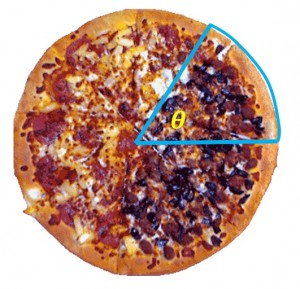Since the arc length is equal to the radius, it must be that the ratio is 1:1.  That makes the central angle one radian.  That’s the angle Feynman was using to describe his errant throw.

Now let’s get rid of the pizza and just look at the angle.  Suppose you increase that angle just a little to pull the crust into a straight line (without changing its length).  You would now have an equilateral triangle.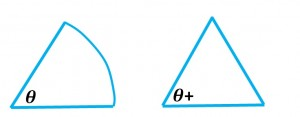That tells us something interesting:  one radian is a little less than the angle of an equilateral triangle.  And since we already knew that the three of the angles of an equilateral triangle (in fact,of any triangle) add up to a straight line, it must take a little more than 3 radians to also add up to a straight line.

How much more?  You already know that too:  π radians = 180 degrees. Right. But maybe now you have a little more of that radian feeling.

THIS JUST IN:

I found this on BusinessInsider.com in an article by Walter Hickey.Nice.

# Angle Measurement for Pizza-Crust Lovers

Whether we measure a right angle to be 90 degrees or 100 grads, either way we are adopting an arbitrary scale.  But when we say that its measure is π/2 radians, we are using a system that is not arbitrary at all.  It is based on a geometric comparison that works a lot like an aspect ratio.

As we said earlier, the aspect ratio of a rectangle is the ratio of length to width.  Intuitively, it is a measure of the “rectangularity”.  Long skinny rectangles have high aspect ratios.  Squares have aspect ratio L/W = 1.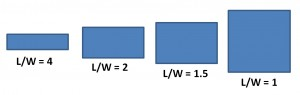One important thing to understand about aspect ratios: they don’t tell you anything about the size of the rectangle.  These two rectangles both have the same aspect ratio (2.5) though one is clearly bigger: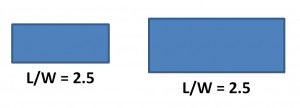Aspect ratios tell you about the shape, not the size.

Also, as long as you measure the length and width with the same units, the aspect ratio is “dimensionless”.  For a given rectangle, you get the same aspect ratio whether you measure your length and width in inches, feet, yards, miles…it doesn’t matter, as long as you use the same units for both measurements.

Onward to Angle Measurement

Here again are a collection of slices all cut from the same pizza but with different central angles: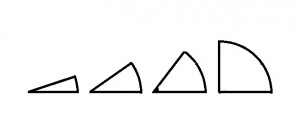And here’s a critical fact to notice about these slices: for pizza of a given radius, the bigger the angle, the more crust you get.  (Many of my mathematical insights involve food. Why is that?)

The crust length is better known as “arc length”.  And now we can define a kind of aspect ratio.  Instead of length-to-width, let’s base this aspect ratio on the arc length and the radius.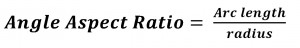The arc length-to-radius ratio is a natural and completely non-arbitrary way to measure the angles.  All of the slices above have the same radius.  So the biggest arc length-to-radius ratio is found on the right where the crust is longest.  And that slice has the biggest central angle.

For example, suppose you cut two slices from a pizza with a radius of 12 inches, one with 6 inches of crust and the other with 9 inches of crust.

We can define the measure of the central angle as the Angle Aspect Ratio.

For the smaller piece…

Angle Aspect Ratio = Arc Length / Radius = 6inches / 12 inches = 1/2.

And for the larger piece…

Angle Aspect Ratio = Arc Length / Radius = 9inches / 12 inches = 3/4.

As expected, the larger Angle Aspect Ratio is associated with the larger central angle.  You got more crust per radius with that angle.

Also, just like aspect ratios for rectangles, it’s the shape and not the size that matters. These two slices have the same angular aspect ratio.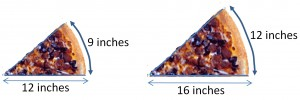The bigger slice has more crust but the crust-to-radius ratio is the same. So both of these angles have the same measure:And yes, the real name for this system is called “radian measure” (and not “angle aspect ratio”, alas).

In this system, the size of a right angle is not an arbitrary 90 degrees or 100 grads.  Instead, it is the ratio of the arc length to the radius.  But we can figure out that value.  We know a full circle has a circumference of 2πr.  So the arc length of a quarter circle must be 2πr ÷ 4 =πr/2.Then, to find the radian measure, we calculate the “angle aspect ratio” (as I stubbornly call it):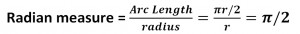And now that we know that a right angle measures π/2 radians, we can also say that

That’s a useful fact if you want to convert back and forth from degrees to radians.  But if all you know is that conversion factor, then you have missed the point. I know that some of my students think of radians the way they think of the metric system: another unfamiliar system you have to learn because math and science teachers insist on it.  But seriously, mathematicians are as lazy as anyone else.  They would never have adopted the system of radian measure if it didn’t have advantages over the more commonly used degree measure.  Some of these advantages don’t show up until you are studying physics and calculus.  But some of them can be seen sooner than that.  Stay tuned…

# Measuring Angles

If you have been following my recent posts, you may now be waiting for an impassioned defense of “completing the square”.  It’s coming soon, with pictures (of course).  But talking about the aspect ratio of a rectangle got me thinking about other ratios that show up in geometry. In particular, I want to look at what we might call the aspect ratio of an angle.  This will lead us to a natural way of describing the size of an angle, one that is less arbitrary than the degree system.  So I have interrupted the regularly scheduled digression for a different digression.

Measuring Angles the Familiar Way

When two rays intersect, we say that they form an angle.  For example, the two rays could be cut along different radii of a pizza, intersecting at the center of the pie.  Depending on how you make your cuts, you will get different sizes for your central angle.  If you are hungrier, you cut a larger central angle.Since angles come in different sizes, we need to have a system for measuring them.  And of course, we have a system that you have known forever.  We call a full circle 360 degrees.  We call a u-turn a “180”, short for 180 degrees.  We call the central angle of a quarter of a pizza a 90-degree angle.  That is also the measure of the angle you find in the corner of every rectangle.  It’s such a familiar system that you may not even realize that it is completely arbitrary.  Who chose 360 as the magic number for a full circle?  (I believe it goes back to the ancient Sumerians, but I haven’t even checked Wikipedia.)  My point is that we didn’t have to do it this way (and we don’t always).

Another way we could measure angles

I’m just saying that this would work too:

Let’s describe the size of an angle by comparing it to a right angle, defining the measure of each angle as a percent of the measure of a right angle.  So for example, the angle made by the diagonal of a square is half the size of a right angle.  You are used to calling that a 45 degree angle, but in our new system, that would be an angle measure of 50 — the diagonal makes 50% of a right angle.Here are some fun facts about our new system:

1. A right angle has measure of 100. (It is 100% of a right angle!)

2. A full rotation has measure 400. (Cut a pizza into 4 quarters.  The four right angles add up to a complete circle.

3. The measures of the angles of a triangle still add up to the same as the measure of a straight line.  They each have a total measure of 200 in this system.

4. Equilateral triangles have 3 angles with the same measure: 200/3 = 66.666…

This seems arbitrary and unfamiliar – compared to our current system which is arbitrary but at least familiar.  But here’s one other fun fact about our new system:

5.  It isn’t new at all!  It’s an existing system.  When you express an angle as a percent of a right angle, you are measuring the angle in “gradians” or “grads”.  This a real thing. It may even be one of the modes on your calculator (but no one ever uses it).

I’ve read that gradians were used in navigation but I have never seen it done.  To me, it feels like a quirk of history that we chose one arbitrary system over another.  There is, however, another way to measure the size of an angle that is not arbitrary at all.  It is a natural way to describe different angles using ratios in a manner much like the way they are used to describe rectangles.  This system has a real name: it’s called “radian measure” (and your calculator has a mode for this as well).  But we can also think of it as the “Angular Aspect Ratio”.   I’ll describe how it works in my next post.

# Rectangles and the Golden Ratio

These may all be digressions, but (as I reassure my students all the time), stay with me.  I’m heading somewhere with all of this.  For now, let’s just keep going.

Here is a rectangle:And, yes, in Your First Book of Shapes or its equivalent, that was the shape on the “Square” page.  But then in geometry class, you learned that the squares are a subset of the rectangles.  They just happen to have equal length and width.  Still, when I say “picture a rectangle”,  you probably don’t picture a square.  So I’ll try again.

Here is a rectangle:OK, that is surely a rectangle and not a square.  But is it what you picture when I say “rectangle”?  I’m guessing that my rectangle is skinnier than the one in your mind.  Some rectangles are more “rectangular-y” than others.  We could say they have higher “rectangularity”.  But is there a way to put a number on that?  Actually, there is.  We can use ratios.

Ratios are perfect for this job.  A ratio is a comparison using division.  To find the ratio of A to B, we calculate A÷B.  The answer tells us how many times bigger A is than B.

So let’s define the “Rectangularity” of a rectangle as the ratio of its length to its width.  In that case, my first rectangle had rectangularity of 1 which made it a square.  And my second rectangle had rectangularity of 12.  Its length was 12 times as great as its width.

We can keep calling this ratio “rectangularity”, a name that I made up, but in fact it already has a name: the length-to-width ratio of a rectangle is called its “aspect ratio”.    And in classical design, there is an aspect ratio that is considered ideal.  It just looks right: not too square-y, not too rectangular-y …just right.    It is called the Golden Ratio.  You can find tons of information about  it on-line so I won’t try to replicate it all here.  I just want to look at one interesting feature of a golden rectangle and show how we can use that feature to calculate the Golden Ratio.It looks nice.  But here’s the interesting property.

Slice off a square on one end…that little rectangle that remains is also a golden rectangle.  And yes, if you slice off the end square of this little rectangle, you get another even littler golden rectangle.Repeat as many times as you like to produce an endless stream of ever-smaller golden rectangles.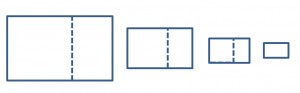Let’s start again.  Suppose the width of the rectangle = 1 and the length = x.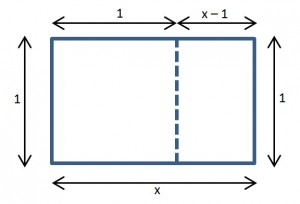Since the ratio  x/1=x, x must also be the value of the golden ratio.  But what is that value?

Well, for the little rectangle, the length is 1 and the width is x-1.  So that rectangle has an aspect ratio of 1/(x-1).  But that aspect ratio is the same as the aspect ratio of the bigger rectangle we started with.  We can write: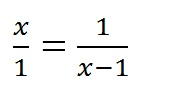To find the value of the golden ratio, we just have to solve that equation.  When you cross-multiply, you get:

x(x-1) = 1

You can distribute to get:

x2 – x = 1

But now what?  If you use trial and error with a calculator, you will soon find that the golden ratio is a little more than 1.6.  But if you want an exact solution, you need to know how to handle quadratic equations.

And that’s what I really want to talk about.  Quadratic equations pop up in physics.  Not every day, but occasionally.  And they usually can’t be factored.  (Neither can the one we have here.)  My students deal with them a variety of ways.  Some grab their TI89s and let the calculator do the work.   Some of them  use the quadratic formula.  Almost nobody “completes the square”.

I admit that I used to make fun of completing the square as a classic piece of cook-book math – all procedure, no understanding.  But I think I was wrong about that.  I wasn’t picturing the procedure geometrically.  And, as I have mentioned in a few other posts, it’s better with pictures.  I’ll explain in the next post.